## Excel DEVSQ function

The DEVSQ function calculates the sum of squares of the deviations from the sample mean.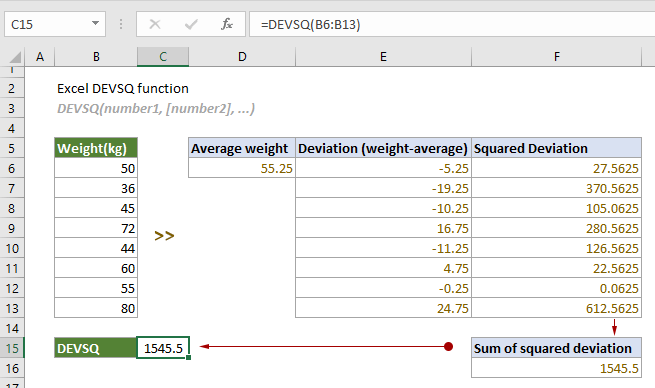#### Syntax

DEVSQ(number1, [number2], ...)

#### Arguments

• Number1 (required): The first number or reference used in the calculation;
• Number2,... (optional): The second and more numbers or references used in the calculation.

#### Remarks

1. There can be up to 255 arguments at a time;
2. Arguments can be:
-- Numbers;
-- Range names, arrays or references that contain numbers.
3. Logical values and text representations of numbers that you directly type in the arguments will be included in the calculation:
-- Logical values: TRUE (1) or FALSE (0);
-- Text representations of numbers: numbers enclosed in double quotation marks such as “2”.
The formula below:
=DEVSQ(1,"2",TRUE,10,8) //The result is 73.2
can be seen as:
=DEVSQ(1,2,1,10,8) //The result is 73.2
4. If the reference or array contains logical values, text, or empty cells, they will be ignored;
5. Cells with zero values will be included in the calculation as well;
6. The #NUM! error occurs when all supplied arguments are nonnumeric;
7. The #VALUE! error occurs if any of the supplied arguments you typed directly in the function are text strings that cannot be interpreted as numbers by Excel;
8. The equation for the DEVSQ function is: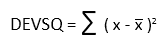Where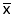is the average of the set of values.

#### Return value

It returns a numeric value.

#### Example

As shown in the screenshot below, there is a list of weights in the range B6:B13. To calculate the sum of squared deviations from their mean, you can apply the DEVSQ function as follows to get it done.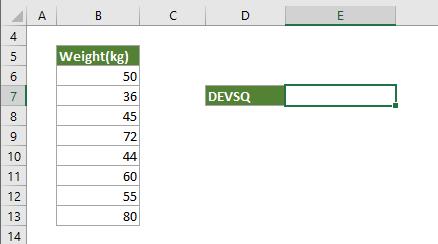Select a cell (E7 in this case), copy or enter the formula below and press Enter to get the result.

=DEVSQ(B6:B13)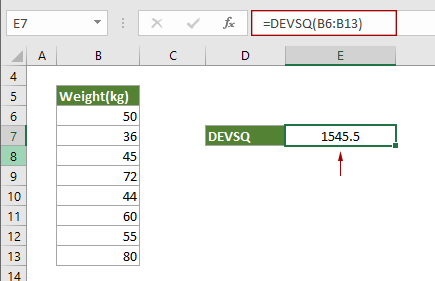Notes: Arguments in the formula above are supplied as a cell range. However, you can change the formula as follows:

1. Directly type numbers as arguments:

=DEVSQ(50,36,45,72,44,60,55,80)

=DEVSQ({50;36;45;72;44;60;55;80})

2. Reference to each cell in the range:

=DEVSQ(B6,B7,B8,B9,B10,B11,B12,B13)

3. If you have created a name for the range, just reference the range name in the formula (supposing the name of the range is Weight):

=DEVSQ(Weight)

#### Related Functions

Excel SUMX2MY2 function
The SUMX2MY2 function returns the sum of the difference of squares of corresponding values in two given arrays.

Excel SUMX2PY2 function
The SUMX2PY2 function returns the sum of squares of corresponding values in two given arrays.

### The Best Office Productivity Tools

#### Kutools for Excel - Helps You To Stand Out From Crowd

 Popular Features: Find, Highlight or Identify Duplicates  |  Delete Blank Rows  |  Combine Columns or Cells without Losing Data  |  Round without Formula ... Super VLookup: Multiple Criteria  |  Multiple Value  |  Across Multi-Sheets  |  Fuzzy Lookup... Adv. Drop-down List: Easy Drop Down List  |  Dependent Drop Down List  |  Multi-select Drop Down List... Column Manager: Add a Specific Number of Columns  |  Move Columns  |  Toggle Visibility Status of Hidden Columns  |  Compare Columns to Select Same & Different Cells ... Featured Features: Grid Focus  |  Design View  |  Big Formula Bar  |  Workbook & Sheet Manager | Resource Library (Auto Text)  |  Date Picker  |  Combine Worksheets  |  Encrypt/Decrypt Cells  |  Send Emails by List  |  Super Filter  |  Special Filter (filter bold/italic/strikethrough...) ... Top 15 Toolsets:  12 Text Tools (Add Text, Remove Characters ...)  |  50+ Chart Types (Gantt Chart ...)  |  40+ Practical Formulas (Calculate age based on birthday ...)  |  19 Insertion Tools (Insert QR Code, Insert Picture from Path ...)  |  12 Conversion Tools (Numbers to Words, Currency Conversion ...)  |  7 Merge & Split Tools (Advanced Combine Rows, Split Excel Cells ...)  |  ... and more

Kutools for Excel Boasts Over 300 Features, Ensuring That What You Need is Just A Click Away...#### Office Tab - Enable Tabbed Reading and Editing in Microsoft Office (include Excel)

• One second to switch between dozens of open documents!
• Reduce hundreds of mouse clicks for you every day, say goodbye to mouse hand.
• Increases your productivity by 50% when viewing and editing multiple documents.
• Brings Efficient Tabs to Office (include Excel), Just Like Chrome, Edge and Firefox.